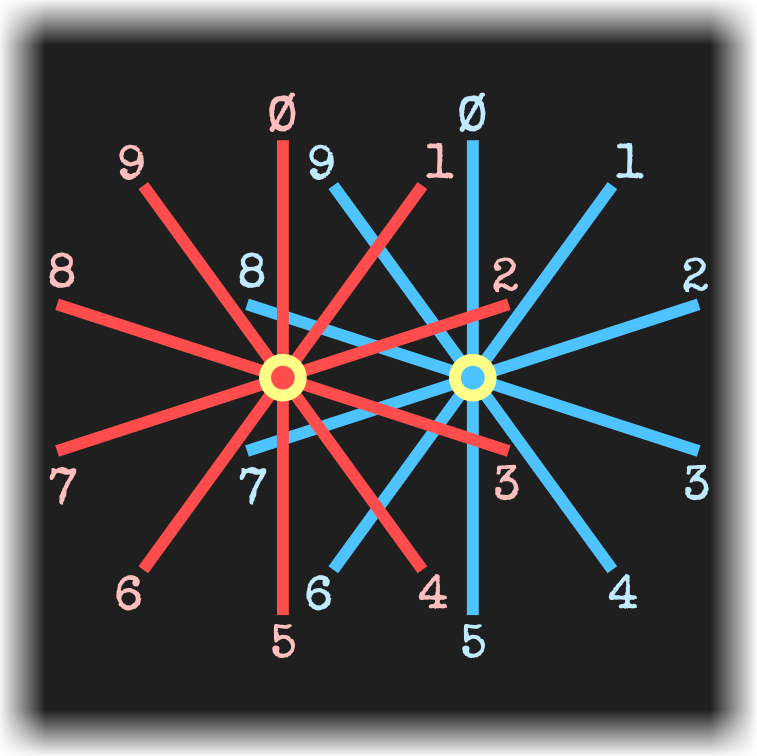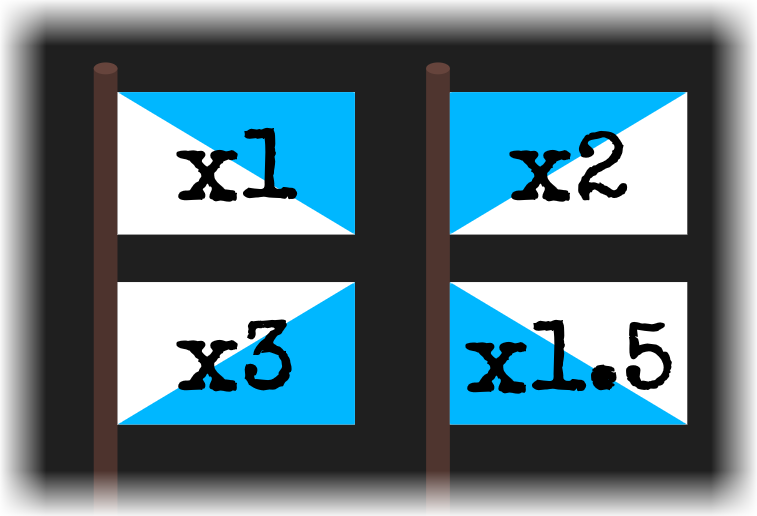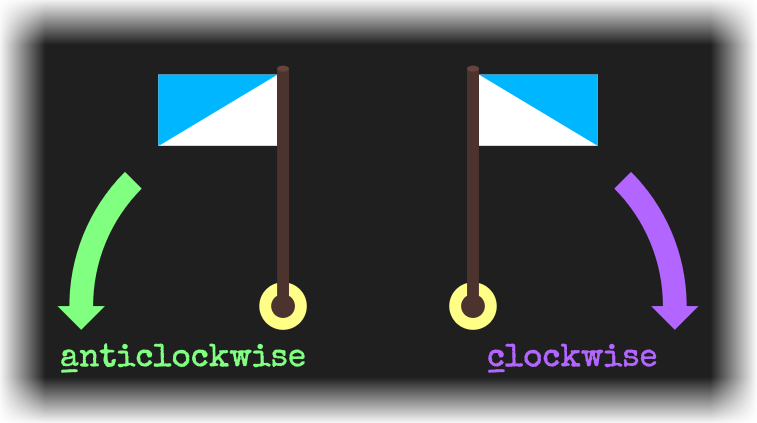﻿ Over Kilo — Keep Talking and Nobody Explodes Module## On the Subject of Over Kilo

Didn’t the 18th Familiar Faces module also have the acronym “O.K.”?

Note: If the flag’s initial arrangement matches the “Letters” or “Numerals” character found in semaphore’s flag alphabet, you are looking at the wrong manual.

An “Over Kilo” module has a field with two flags, each pointed in one of ten directions. Above the field are two round buttons with a greater-than sign (>) and a less-than sign (<), and a square button reading “OK”.

Every “slide” will use the directions of the two masts to represent a 2-digit value, the directions of the two flags to represent a bonus, and the pattern on the flags to represent the permanent multiplier, which will be the same on both flags and will never change during the module. In order to determine the:

• value: Use the directions of the masts in Diagram N, where the left axle (in red) represents the digit in the tens place, and the right axle (in blue) represents the digit in the ones place.
• bonus: Use the direction the flags are pointing relative to their masts in Table A1, where C is clockwise and A is anticlockwise. Diagram A2 provides an example of both.
• permanent multiplier: Use the pattern on the flags (when properly oriented to pointing up and clockwise) in Diagram M.

Compare the current slide’s value to the previous slide’s value. If the current value is greater than the previous value, press the greater-than sign. If the current value is less than the previous value, press the less-than sign. On the first slide, pressing either round button is valid.

Track the total sum of every value and bonus displayed. If at any point the total sum multiplied by the permanent multiplier returns a number greater than one thousand, press the “Over Kilo” (O.K.) button, disregarding any other instructions.

If the total sum multiplied by the permanent multiplier has yet to total above one thousand by the eighth slide, pressing the correct round button will automatically defuse the module.

Incorrectly pressing any button will result in a strike. Correctly pressing the “Over Kilo” button will defuse the module.

### Diagram N### Table A1